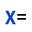## Display Assignment

Adds or removes the name of the current cell from the rendered equation. This name is derived from either the 'Name' assigned to the cell being rendered orfrom the text in the cell immediately to the left of it.

### Appearance

Text (but not formulae) in the cell immediately to the left of the selected equation is added to the front of the equation with an operator separating it from the main equation. The default operator placed between the equation is '=', but a variety of alternatives can also be used as they appear at the end of the selected cells label:

Cell TextOutput
a==
a<=
a>=
a!=
a=>
a~
a~=
a~==
a==>

### Usage

For existing rendered equations the Display Assignment button will turn on/off this feature for all selected equations.

Display Assignment can be used on its own to render text (usually in LaTeX format) within a cell. To avoid confusion, the original text is hidden by changing its colour to match the background. The rendered text will aligned to the right of the selected cell.

Important: The easiest way to restore the original text colour is to select the rendered equation and click the Erase button (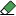).

For example:

Cell LaTeX Output
\alpha+\beta
\int_{0}^{x}13x\cdot dx
f(x)=\int_{a}^{b}K(x,t)\phi(t)dt
f\xi =\int_{-\infty}^{x}f(x)e ^{-2\pi ix\xi }dx

For help writing LaTeX, use the CodeCogs Online Equation Editor (also accessible from the LaTeX button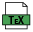in the toolbar) and copy the LaTeX text created back into the cell in Excel.

Ctrl Key: The default state of the Display Assignment button can be configured either by selecting an empty cell and clicking the button or clicking the button with the control-key selected. The button will then appear as permanently selected or deselected. Thereafter, all new rendered equations will adopt this setting.

### Example 6

Download the example provided and follow the steps below:

• Cells C5 and C8 are selected, and rendered using Cell Name.
• If Display Assignment (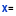) is not already on, select the button while formulas or rendered equations are still selected.
• The rendered equations are shown to the right of the selected formulae, with their assigned name (or label).
•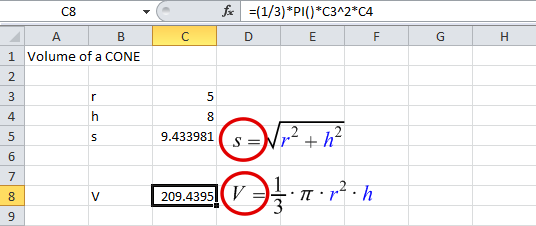Example 2: Style: Excel Buttons: